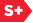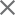### Proceedings Paper

Spectral radius of sets of matrices
Author(s): Mohsen Maesumi
Format Member Price Non-Member Price
PDF \$17.00 \$21.00

Paper Abstract

Spectral radius of sets of matrices is a fundamental concept in studying the regularity of compactly supported wavelets. Here we review the basic properties of spectral radius and describe how to increase the efficiency of estimation of a lower bound for it. Spectral radius of sets of matrices can be defined by generalizing appropriate definitions of spectral radius of a single matrix. One definition, referred to as generalized spectral radius, is constructed as follows. Let (Sigma) be a collection of m square matrices of same size. Suppose Ln((Sigma) ) is the set of products of length n of elements (Sigma) . Define pn((Sigma) ) equals maxA(epsilon Ln [p(A)]1/n where p(A) is the usual spectral radius of a matrix. Then the generalized spectral radius of (Sigma) is p((Sigma) ) equals lim supnyields(infinity )pn((Sigma) ). The standard method for estimating p((Sigma) ), through pn((Sigma) ), involves mn matrix calculations, one per each element of Ln((Sigma) ). We will describe a method which reduces this cost to mn/n or less.

Paper Details

Date Published: 11 October 1994
PDF: 9 pages
Proc. SPIE 2303, Wavelet Applications in Signal and Image Processing II, (11 October 1994); doi: 10.1117/12.188808
Show Author Affiliations
Mohsen Maesumi, Lamar Univ. (United States)

Published in SPIE Proceedings Vol. 2303:
Wavelet Applications in Signal and Image Processing II
Andrew F. Laine; Michael A. Unser, Editor(s)